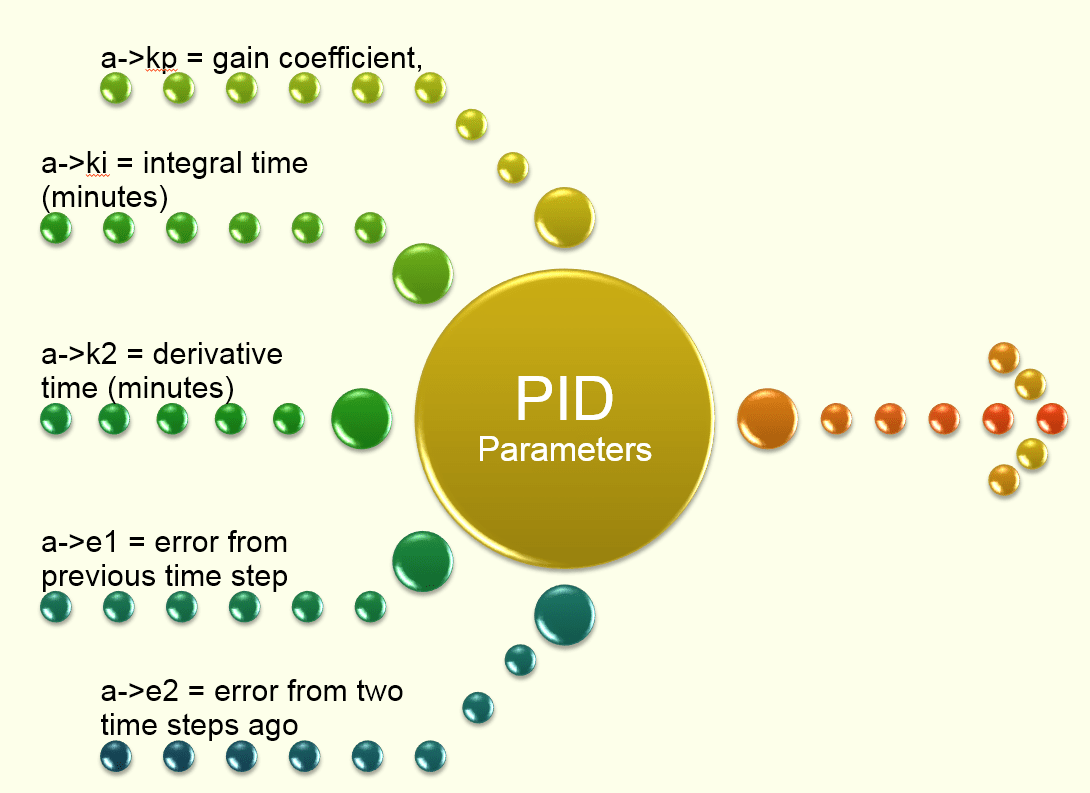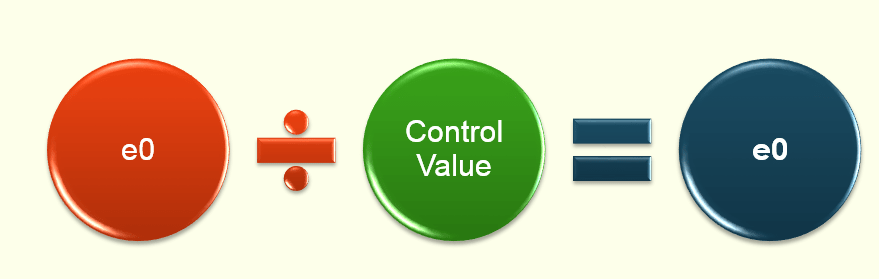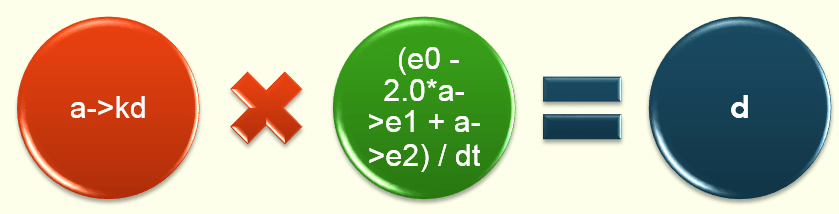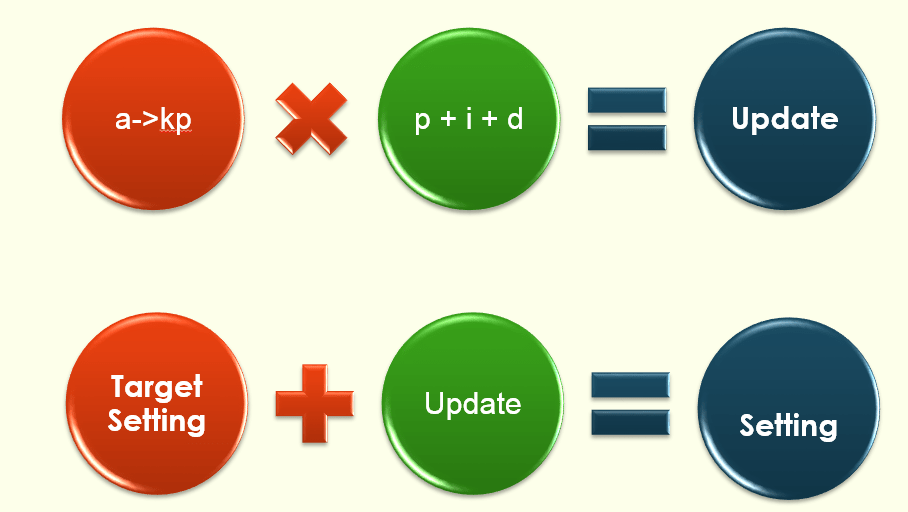#INFOSWMM

# Annotated PID Controller in SWMM5 and InfoSWMM

How does a PID controller work in  InfoSWMM  and SWMMM 5 – this also applies to InfoSWMM SA

double getPIDSetting(struct TAction* a, double dt)   //  at each time  step find  the PID control  changes for the  current  time step dt
// Input: a = an action object
// dt = current time step (days)
// Output: returns a new link setting
// Purpose: computes a new setting for a link subject to a PID controller.// Note: a->kp = gain coefficient,
// a->ki = integral time (minutes)
// a->k2 = derivative time (minutes)
// a->e1 = error from previous time step
// a->e2 = error from two time steps ago
{
double e0, setting;
double p, i, d, update;
double tolerance = 0.0001;

// — convert time step from days to minutes
dt *= 1440.0;

// — determine relative error in achieving controller set point

// Or how close are we to the set point?e0 = SetPoint – ControlValue;
if ( fabs(e0) > TINY )
{
if ( SetPoint != 0.0 ) e0 = e0/SetPoint;

// now alter the  value of e0else e0 = e0/ControlValue;
}

// — reset previous errors to 0 if controller gets stuck
if (fabs(e0 – a->e1) < tolerance)
{
a->e2 = 0.0;
a->e1 = 0.0;
}

// — use the recursive form of the PID controller equation to
// determine the new setting for the controlled link

Here is a view of the p, i and d PID internal  parameters https://www.wikiwand.com/en/PID_controllerA block diagram of a PID controller in a feedback loop

p = (e0 – a->e1);ki, id,  kp are  user  inputs  in InfoSWMM and SWMM5 or from the  EPA SWMM  5 Help  Fileif ( a->ki == 0.0 ) i = 0.0;
else i = e0 * dt / a->ki;d = a->kd * (e0 – 2.0*a->e1 + a->e2) / dt;update = a->kp * (p + i + d);
if ( fabs(update) < tolerance ) update = 0.0;// — update previous errors
a->e2 = a->e1;
a->e1 = e0;

// — check that new setting lies within feasible limits

// If  the link  is not a pump then the  setting must be between 0 and 1
if ( setting < 0.0 ) setting = 0.0;
if (Link[a->link].type != PUMP && setting > 1.0 ) setting = 1.0;
return setting;}